Next: The simple pendulum Up: Oscillatory motion Previous: Simple harmonic motion

## The torsion pendulum

Consider a disk suspended from a torsion wire attached to its centre. See Fig. 96. This setup is known as a torsion pendulum. A torsion wire is essentially inextensible, but is free to twist about its axis. Of course, as the wire twists it also causes the disk attached to it to rotate in the horizontal plane. Letbe the angle of rotation of the disk, and let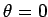correspond to the case in which the wire is untwisted.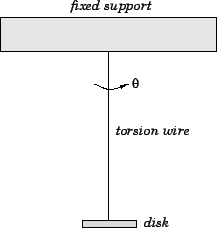Any twisting of the wire is inevitably associated with mechanical deformation. The wire resists such deformation by developing a restoring torque,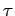, which acts to restore the wire to its untwisted state. For relatively small angles of twist, the magnitude of this torque is directly proportional to the twist angle. Hence, we can write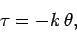(518)

where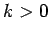is the torque constant of the wire. The above equation is essentially a torsional equivalent to Hooke's law. The rotational equation of motion of the system is written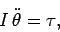(519)

where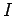is the moment of inertia of the disk (about a perpendicular axis through its centre). The moment of inertia of the wire is assumed to be negligible. Combining the previous two equations, we obtain(520)

Equation (520) is clearly a simple harmonic equation [cf., Eq. (504)]. Hence, we can immediately write the standard solution [cf., Eq. (505)]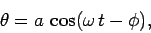(521)

where [cf., Eq. (507)](522)

We conclude that when a torsion pendulum is perturbed from its equilibrium state (i.e.,), it executes torsional oscillations about this state at a fixed frequency,, which depends only on the torque constant of the wire and the moment of inertia of the disk. Note, in particular, that the frequency is independent of the amplitude of the oscillation [providedremains small enough that Eq. (518) still applies]. Torsion pendulums are often used for time-keeping purposes. For instance, the balance wheel in a mechanical wristwatch is a torsion pendulum in which the restoring torque is provided by a coiled spring.Next: The simple pendulum Up: Oscillatory motion Previous: Simple harmonic motion
Richard Fitzpatrick 2006-02-02